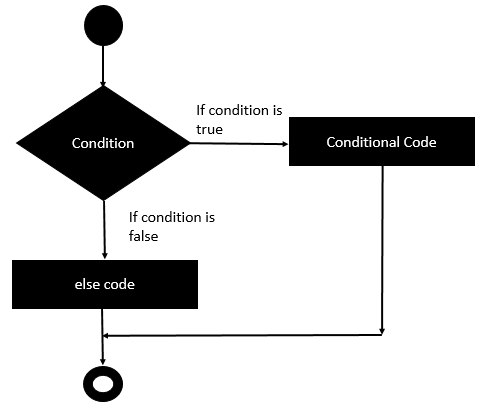# Rexx - If else statement

The next decision-making statement is the if-else statement. An if statement can be followed by an optional else statement, which executes when the Boolean expression is false.

## Syntax

The general form of this statement in Rexx is as follows. −

```if (condition) then
do
#statement1
#statement2
end
else
do
#statement3
#statement4
end
```

In Rexx, the condition is an expression which evaluates to either true or false. If the condition is true, then the subsequent statements are executed. Else if the condition is evaluated to false, then the statements in the else condition are evaluated.

## Flow Diagram

The flow diagram of the if-else statement is as follows −From the above diagram, it can be noted that we have two code blocks. One gets executed if the condition is evaluated to true and the other if the code is evaluated to false.

The following program is an example of the simple if-else expression in Rexx.

## Example

```/* Main program */
i = 50
if (i < 10) then
do
say "i is less than 10"
end
else
do
say "i is greater than 10"
end
```

The output of the above code will be −

```i is greater than 10
```
rexx_decision_making.htm